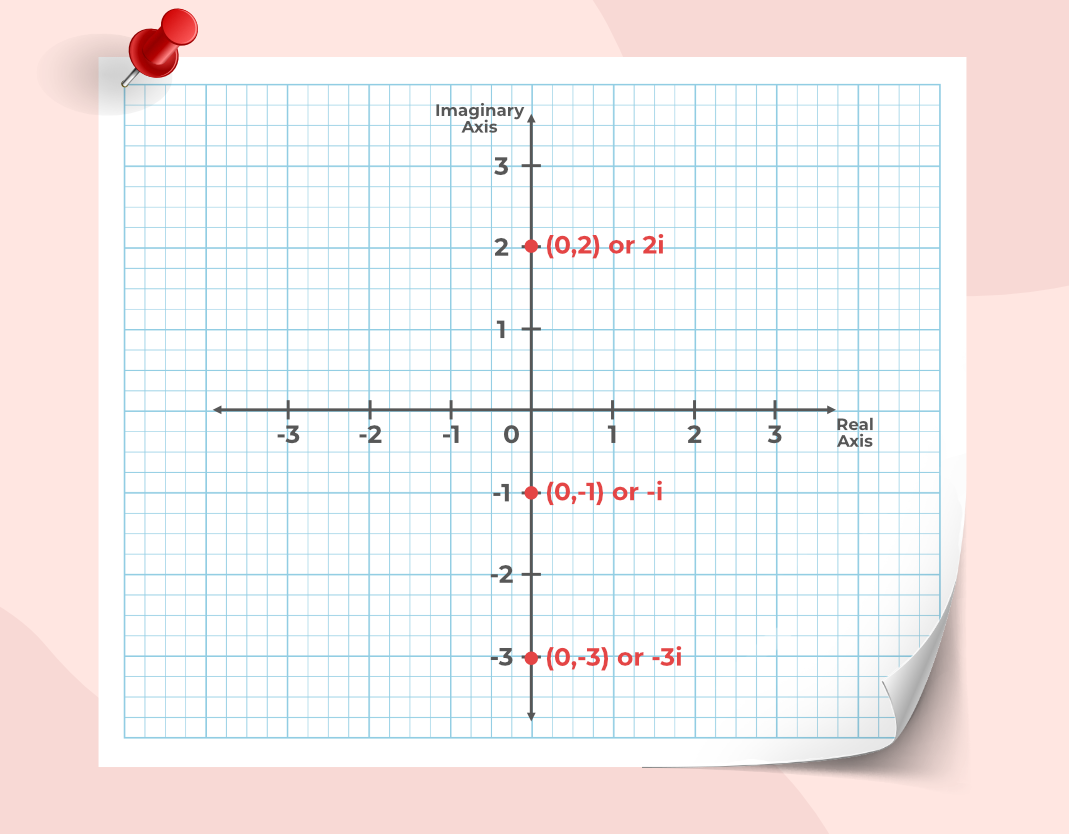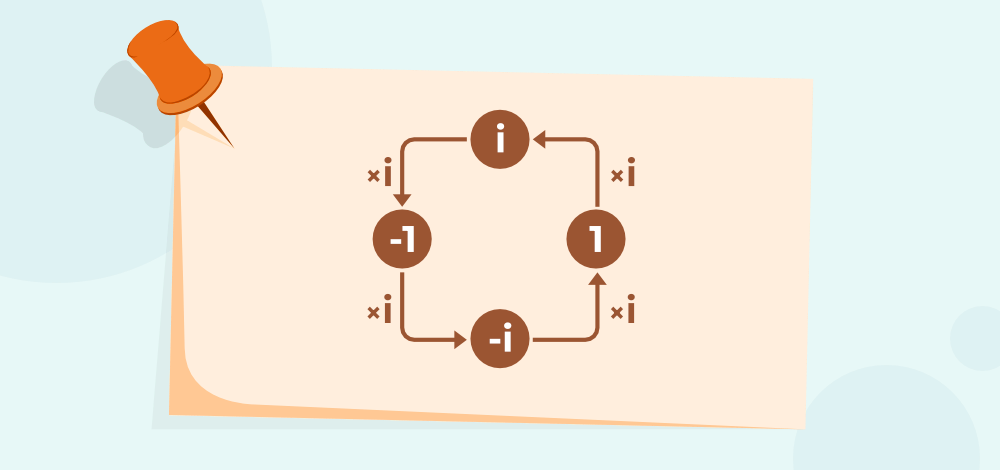Open In App
Related Articles
• CBSE Class 11 Maths Notes
• CBSE Class 11 Maths Formulas
• NCERT Solutions for Class 11 Maths
• RD Sharma Class 11 Solutions for Maths

# Imaginary Numbers

Imaginary numbers are numbers as the name suggests are the number that is not real numbers. All the numbers real and imaginary come under the categories of complex numbers. Imaginary numbers are very useful in solving quadratic equations and other equations whose solutions can not easily be found using general rules. For example, the solution of x2 + x + 1 = 0 can easily be calculated using imaginary numbers.

## Imaginary Numbers Definition

The number whose square results in negative results is called an Imaginary number i.e. the square root of negative numbers is called an imaginary number. They are called imaginary numbers as we cannot associate them with any real-life examples.

They are represented by “i” and are pronounced as iota at its value is,

i = √-1

For example, 2 + 6i is a complex number.

where
2 is the real number part
6i is the imaginary part

Here, the square of real part 2 results in 4 i.e. 22 = 4, and the square of imaginary part results in a negative value, i.e. (6i)2 = -36

The image given below shows the value of i.## What is a Complex Number?

All the number known to us comes under the category of Complex numbers i.e. complex numbers are defined as the superset of all the numbers. We can define complex numbers as a set of numbers containing both real and imaginary numbers.

The standard form of the complex number is a + bi

where
a and b are real numbers
i is an imaginary unit

• Examples of Real Numbers are 2, 3, -4, -5, etc.
• Examples of Imaginary Numbers are i, 11i, -2/3i, etc.
• Examples of Complex Numbers are 11 + 4/3 i, 2 – 3i, 0 + 11i, 3 + 0i, etc.

## History of Imaginary Numbers

The credit for familiarising the concept of imaginary numbers goes to the Greek mathematician called Hero of Alexandria but this concept of further completed in 1572, by an Italian mathematician named Gerolamo Cardano. Imaginary numbers are very helpful in finding the square roots of negative numbers and also help in finding the solution to quadratic equations and other.

## Geometrical Interpretation of Imaginary Numbers

We usually represent a complex number a+bi by a point (a, b) in the Argand plane. For example, a complex number 5-6i is represented by the point (5, -6) on the Argand plane.

Imaginary numbers in the form of bi (written as 0 + bi) are represented by the point (0, b) on the plane, and hence it is a point on the vertical axis (imaginary axis). Thus, the imaginary numbers always lie on the vertical axis of an Argand plane.

Here are a few examples of the imaginary numbers shown in the image given below.## Rules of Imaginary Number

Some of the important rules of Imaginary numbers are,

• Imaginary numbers always exist in conjugate pairs i.e. for example if the complex number a + ib exists then its conjugate pair a – ib also exists.
• Associating imaginary numbers with real values is impossible.
• The square of the imaginary numbers results in a negative number which is the polar opposite of the real numbers, i.e. i2 = -1.

## Powers of i

We know that i2 = -1 and using the power rules other powers of I can also be easily calculated.

• i = √-1
• i2 = -1
• i3 = i2×i = -i
• i4 = (i2)2 = (-1)2 = 1
• i5 = i4×i = i

On observing clearly we can see a pattern, there is a cycle of  -i, 1, i… after power 2 thus,

• i4n-1= -i
• i4n = 1
• i4n+1= i

The image given below explains the multiple of “i” with various numbers.## Operations on Imaginary Numbers

We can perform basic arithmetic operations such as

• Subtraction
• Multiplication
• Division

on the imaginary numbers so now let us discuss these operations on imaginary numbers in detail below,

Now take two complex numbers as a + bi and c + di then,

The addition of imaginary numbers can easily be achieved by using the basic rule of addition, i.e.

For two numbers, a+bi, and c+di when the addition is performed, then the real parts and the imaginary parts are added separately and then simplified.

Example: Add (3 + 11i) and (4 – 5i)

Solution:

(3 + 11i) + (4 – 5i) = (3 + 4) + (11i – 5i)

= 7 + 6i

### Subtraction of Imaginary Numbers

The subtraction of imaginary numbers can easily be achieved by using the basic rule of subtraction, i.e.

For two numbers, a+bi, and c+di when the subtraction is performed, then the real parts and the imaginary parts are subtracted separately and then simplified.

Example: Subtract (3 + 11i) and (4 – 5i)

Solution:

(3 + 11i) – (4 – 5i) = (3 – 4) + (11i + 5i)

= -1 + 16i

### Multiplication of Imaginary Numbers

The multiplication of imaginary numbers can easily be achieved by using the basic rule of multiplication and the distributive property of multiplication, i.e.

For two numbers, a+bi, and c+di the multiplication is explained with the help of the following example.

Example: Multiplying (3 + 11i) and (4 – 5i)

Solution:

(3 + 11i) × (4 – 5i) = (3 × 4) – (3 × 5i) + (11i × 4) – (11i × 5i)

= 12 – 15i + 44i – 55i2

= 12 + 29i -55(-1)   {as i2 = -1}

= 12 + 55 + 29i

= 67 + 29i

### Division of Imaginary Numbers

The division of imaginary numbers can easily be achieved by using the basic rule of division and finding the conjugate of the imaginary numbers.

For two numbers, a+bi, and c+di the division is explained with the help of the following example.

Example: Divide (3 + 11i) and (4 – 5i)

Solution:

= (3 + 11i) / (4 – 5i)

Multiplying the conjugate of the denominator (4 + 5i) to both numerator and denominator we get,

= (3 + 11i) / (4 – 5i) × (4 + 5i) / (4 + 5i)

= {(3 + 11i) × (4 + 5i)} / {(4 – 5i) × (4 + 5i)}

= (12 + 15i + 44i + 55i2) / (16 + 20i – 20i – 25i2)

= (12 – 55 + 59i) / (16 + 25)

= (- 43 + 59i) / 41

## Principal Square Root of Number

The principal Square Root of a non-negative real number is the non-negative square root.

Example:The principal square root of a × b can not be broken down into the principal square root of a × principal square root of b if both a and b are negative.Principal Square Root of -1: The imaginary unit is defined as i2 = −1. Using this notation, we can think of i as the square root of −1, but we also have (−i)2 = i2 = −1 and so −i is also a square root of −1. But by convention, the principal square root of −1 is i, or more generally, if x is any non-negative number, then the principal square root of −x is:Also, Check

## Solved Examples on Imaginary Numbers

Example 1: Evaluate the square root of -121.

Solution:

√(-121) = √(-1 × 121)

= √(-1) × √(121)      {we know that √(-1) = 1}

= i × (±11)

= ±11i

Example 2: Evaluate the square root of -(1/9).

Solution:

√(-1/9) = √(-1 × 1/9)

= √(-1) × √(1/9)      {we know that √(-1) = 1}

= i × (±1/3)

= ±1/3i

Example 3: Solve the imaginary number i5

Solution:

Given Imaginary Number i5

i5 = i2 × i2 × i

i5 = -1 × -1 × i      (i2 = -1)

i5 = 1 × i

i5 = – i

Therefore, i5 is i.

## FAQs on Imaginary Numbers

### Question 1: What are Imaginary Numbers?

The numbers which are not real numbers are called imaginary numbers. They are called imaginary numbers because they can not be associated with any real-life examples. They are represented by “i” called iota and its value is, i = √(-1). Examples 6i, -2i. etc.

### Question 2: What is the value of ‘i’ in Mathematics?

“i” is also called iota in mathematics and its value is defined to be √-1. It is the unit of imaginary numbers and the value of its power are,

• i = √-1
• i2 = -1
• i4 = 1

### Question 3: What is the difference between Imaginary Numbers and Real Numbers?

Imaginary numbers are the numbers whose basic unit is “i” called iota they are widely useful in solving complex equations but their real examples are not easily observed, whereas real numbers are the numbers which are associated with various things such as counting, arranging different values and others.

• Examples of imaginary numbers are 3i, 11i, etc.
• Examples of real numbers are 3, 11, etc.